Find the 11

Find the quotient of 229.12 and 12.32

Result

q =  18.597

Solution:Leave us a comment of this math problem and its solution (i.e. if it is still somewhat unclear...):Be the first to comment!To solve this verbal math problem are needed these knowledge from mathematics:

Need help calculate sum, simplify or multiply fractions? Try our fraction calculator.

Next similar math problems:

1. Typing course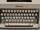Before taking a typing course, Terrence could type 39 words per minute. By the end of the course, he was able to type 68 words per minute. Find the percent increase.
2. DivideDivide the number 72 in the ratio 7: 2 and calculate the ratio of the numbers found in this order and write down as decimal.
3. Percent calculationCalculate 61% if 85% is 38.
4. AlmondsRudi has 4 cups of almonds. His trail mix recipe calls for 2/3 cup of almonds. How many batches of trail mix can he make?
5. Percent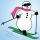From 99 children who participated in the ski course were 23 excellent skiers, 13 good and 20 average and the rest were beginners. Calculate this data in percentages.
6. Determine the number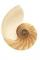Determine the number x that ?.
7. To improper fractionChange mixed number to improper fraction a) 1 2/15 b) -2 15/17
8. FractionDetermine for what x fraction ?:
9. Cleaning windowsCleaning company has to wash all the windows of the school. The first day washes one-sixth of the windows of the school, the next day three more windows than the first day and the remaining 18 windows washes on the third day. Calculate how many windows ha
10. Equation with xSolve the following equation: 2x- (8x + 1) - (x + 2) / 5 = 9
11. FractionsThree-quarters of an unknown number are 4/5. What is 5/6 of this unknown number?
12. UN 1If we add to an unknown number his quarter, we get 210. Identify unknown number.
13. Bag of peanuts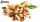Joe eat 1/3 of a bag of peanuts, mark eat 1/4 of the remaining in the bag of peanuts, Alvin eat 1/2 of the remaining bag of peanuts, peter eat 10 peanuts, there are 71 peanuts left. Hon many peanuts were in the bags?
14. InqualitySolve inequality: 3x + 6 > 14
15. Equation with fractionsSolve equation: ? It is equation with fractions.
16. Arble bagA marble bag sold by Rachel's Marble Company contains 5 orange marbles for every 6 green marbles. If a bag has 35 orange marbles, how many green marbles does it contain?
17. Strange x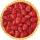For what x is true ??